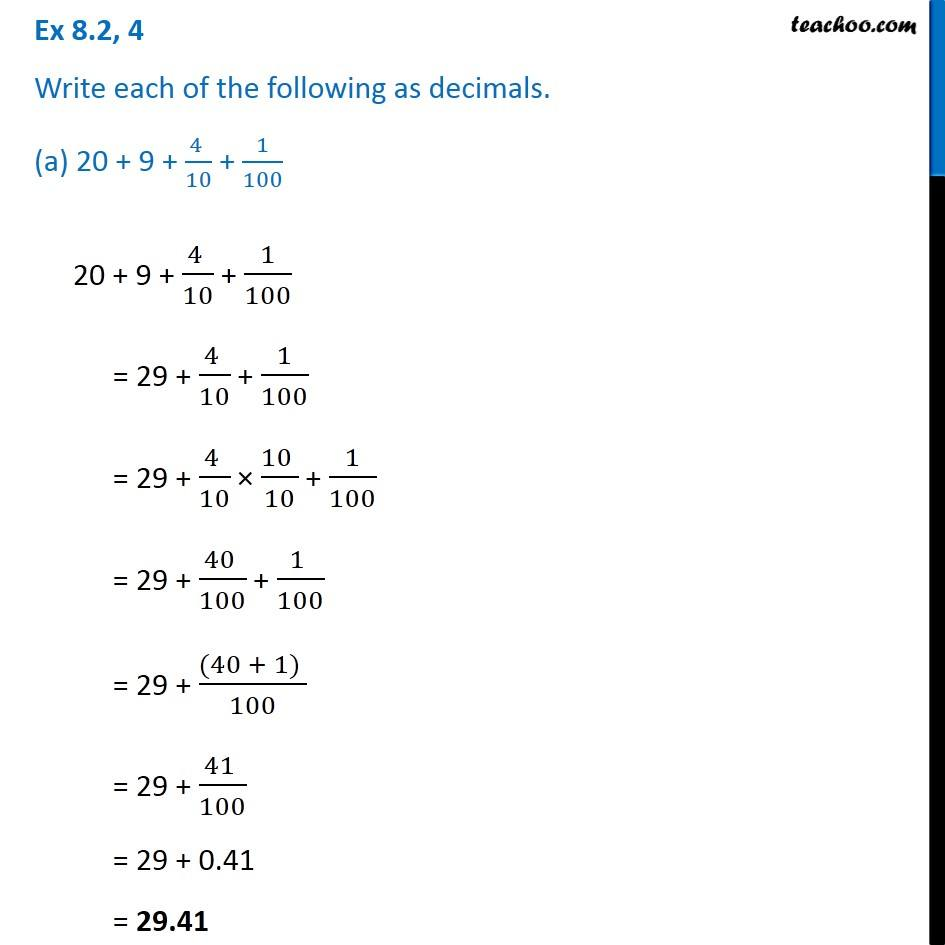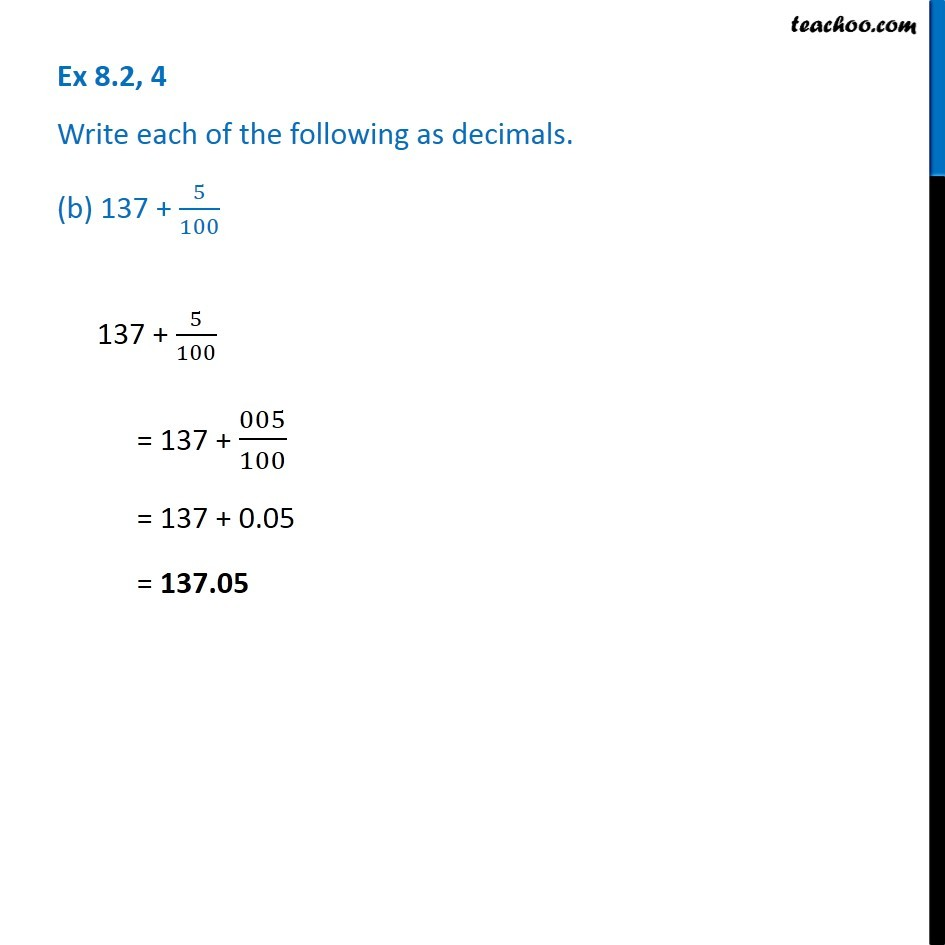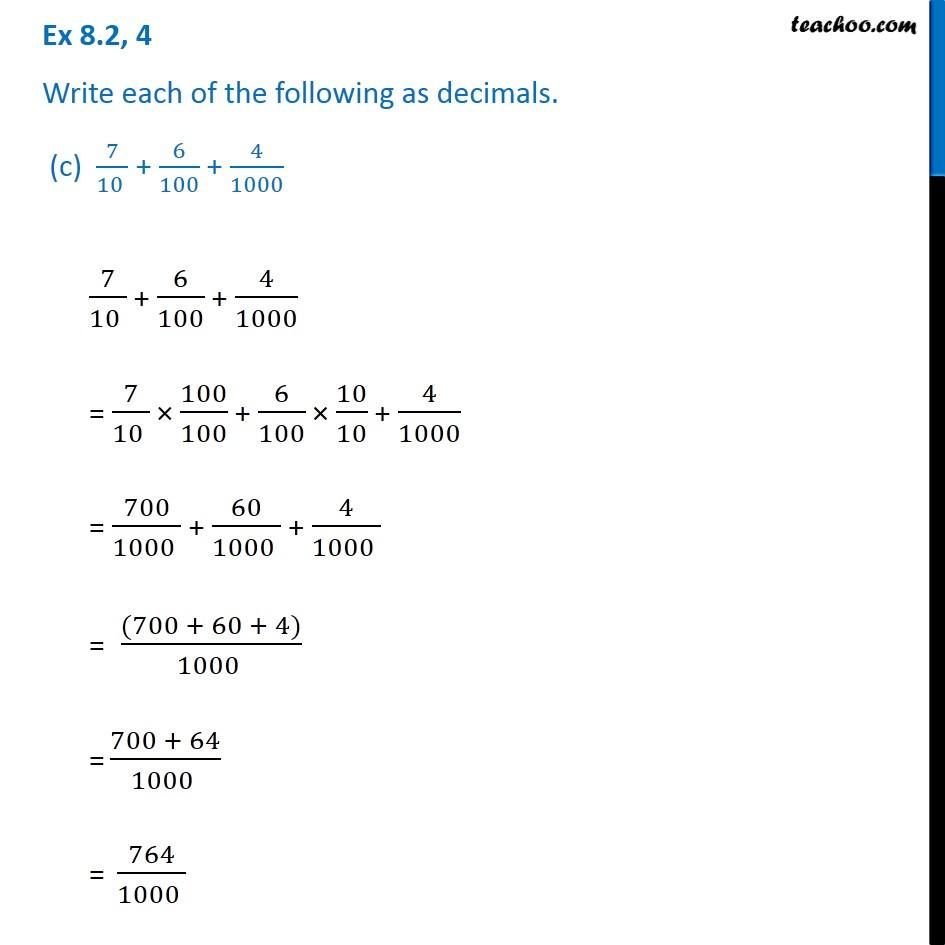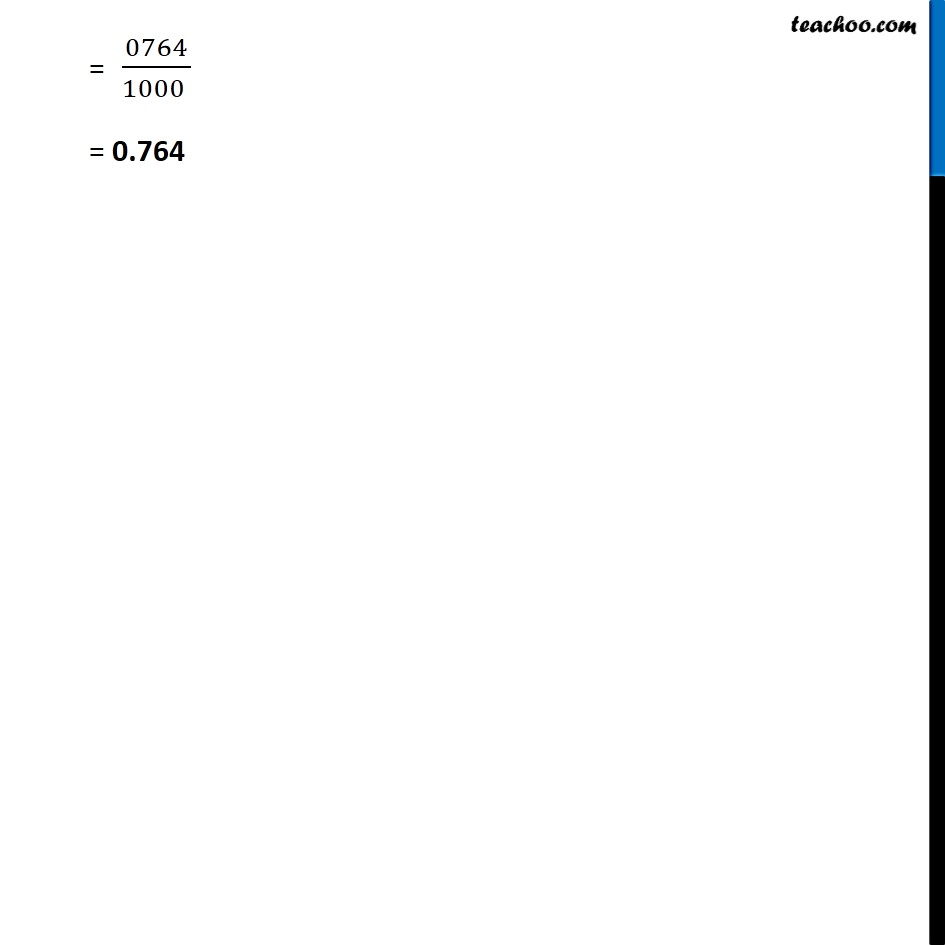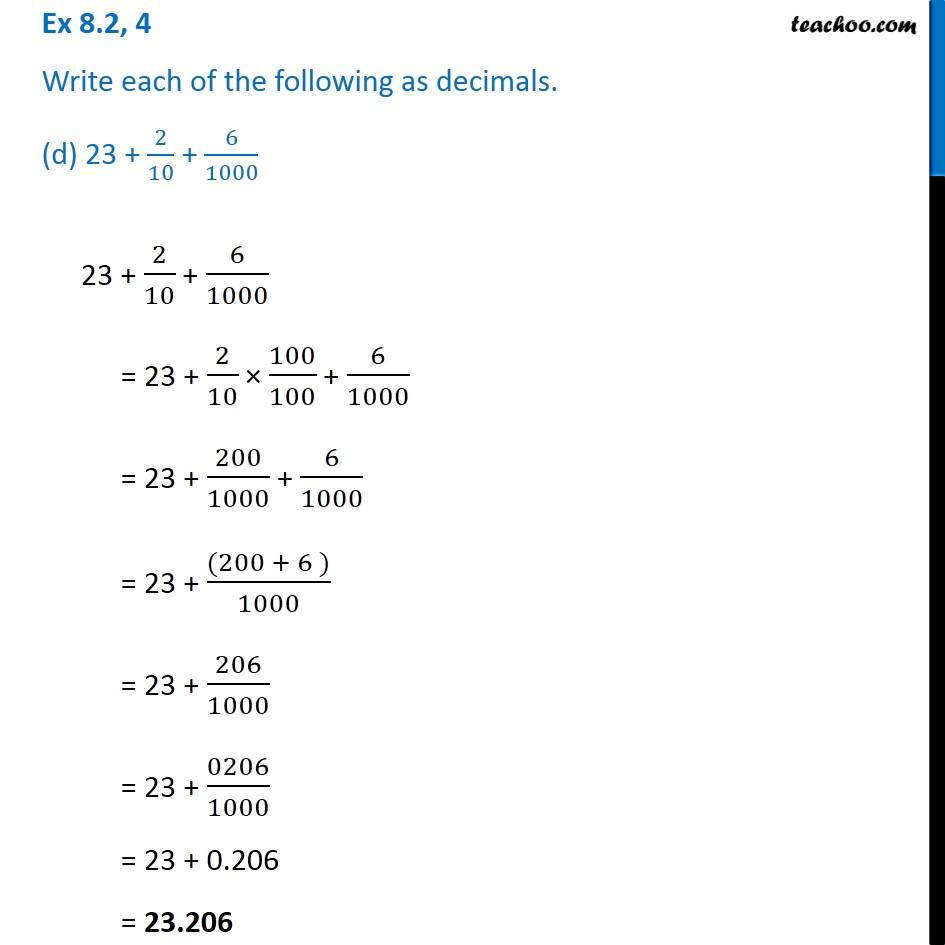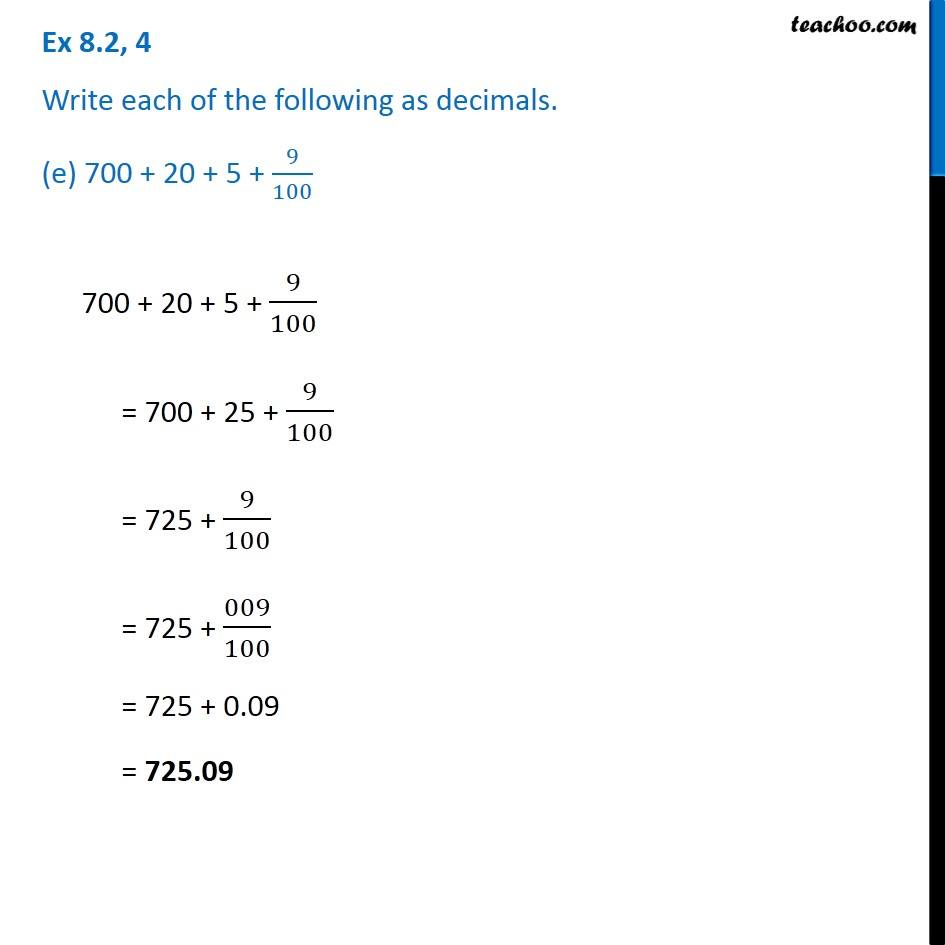Subscribe to our Youtube Channel - https://you.tube/teachoo

1. Chapter 8 Class 6 Decimals
2. Serial order wise
3. Ex 8.2

Transcript

Ex 8.2, 4 Write each of the following as decimals. (a) 20 + 9 + (4 )/10 + 1/100 20 + 9 + (4 )/10 + 1/100 = 29 + (4 )/10 + 1/100 = 29 + (4 )/10 (10 )/10 + 1/100 = 29 + (40 )/100 + (1 )/100 = 29 + ((40 + 1) )/100 = 29 + (41 )/100 = 29 + 0.41 = 29.41 Ex 8.2, 4 Write each of the following as decimals. (b) 137 + 5/100137 + 5/100 = 137 + 005/100 = 137 + 0.05 = 137.05 Ex 8.2, 4 Write each of the following as decimals. (c) 7/(10 ) + 6/100 + 4/1000 7/(10 ) + 6/100 + 4/1000 = 7/(10 ) 100/100 + 6/100 10/10 + 4/1000 = 700/(1000 ) + 60/(1000 ) + 4/(1000 ) = ((700 + 60 + 4))/(1000 ) = (700 + 64)/(1000 ) = 764/(1000 ) = 0764/(1000 ) = 0.764 Ex 8.2, 4 Write each of the following as decimals. (d) 23 + 2/10 + 6/1000 23 + 2/10 + 6/1000 = 23 + 2/10 100/100 + 6/1000 = 23 + 200/1000 + 6/1000 = 23 + ((200 + 6 ))/1000 = 23 + 206/1000 = 23 + 0206/1000 = 23 + 0.206 = 23.206 Ex 8.2, 4 Write each of the following as decimals. (e) 700 + 20 + 5 + 9/100 700 + 20 + 5 + 9/100 = 700 + 25 + 9/100 = 725 + 9/100 = 725 + 009/100 = 725 + 0.09 = 725.09

Ex 8.2

Chapter 8 Class 6 Decimals
Serial order wise